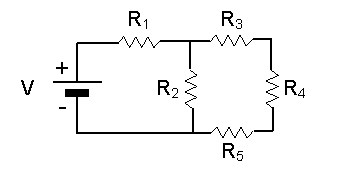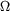# Kirchhoff's Rule Queston

## Homework Statement[/B]
Consider the circuit above. You are given the following measured values:
V = 1.5 V
R1 = 100R2 = 100R3 = 50R4 = 50R5 = 50## Homework Equations

Kirchhoff's Loop Rule and Junction Rule

## The Attempt at a Solution

I tried setting this up using the junction and loop rule.
At the first junction (between R1 and R3), I have I1 = I2 + I3. At the second junction, after R5, I have I5 + I2 = Io (Io is the current entering the battery again).
I do not understand what the loop rule should be written as and then how to put it all together. Please help!

ehild
Homework Helper
Your I5 and I3, and also Io and I1 are related - how?

What does the Loop Rule state?

The loop rule states that the sum of the potential differences around the circuit is 0. Is I3 = -I5? And also I1 = -Io?

ehild
Homework Helper
The loop rule states that the sum of the potential differences around the circuit is 0. Is I3 = -I5? And also I1 = -Io?
The loop rule states that the sum of potential differences is zero around a loop.

I3 = - I5 would mean that the current flows into R4 from both sides and the charges accumulate there. Is it possible? The current flows through a resistor, enters at one terminal and leaves at the other. What do you know about the current flowing through resistors connected in series?